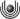# Prof. Dr. Martin Stynes: A finite difference method for the time-fractional heat equation

## Kolloquium der Fakultät für Mathematik und Informatik

Termin: 21.11.2016

Loading the player ...

Abstract:

An introduction to fractional derivatives and their properties is presented first. Then we consider a time-fractional version of the classical parabolic heat equation. The solution of such a problem is shown in general to have a weak singularity near the initial time. A new analysis of a standard finite difference method for the problem is given, taking into account this initial singularity. This analysis encompasses both uniform meshes and meshes that are graded in time, and shows clearly how the grading of the mesh affects the order of convergence of the difference scheme, so one can choose an optimal mesh grading. Numerical results are presented that confirm the sharpness of the error analysis.

videostreaming | 22.11.2016FernUniversität in Hagen, ZMI, 58084 Hagen, E-Mail: viedeostreaming(at)fernuni-hagen.de